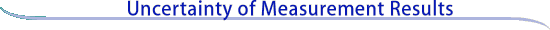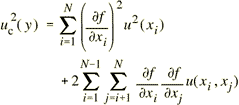Combining uncertainty components

Calculation of combined standard uncertainty
The combined standard uncertainty of the measurement result y, designated by uc(y) and taken to represent the estimated standard deviation of the result, is the positive square root of the estimated variance uc2(y) obtained from(6)

Equation (6) is based on a first-order Taylor series approximation of the measurement equation Y = f(X1, X2, . . . , XN) given in equation (1) and is conveniently referred to as the law of propagation of uncertainty. The partial derivatives of f with respect to the Xi (often referred to as sensitivity coefficients) are equal to the partial derivatives of f with respect to the Xi evaluated at Xi = xi; u(xi) is the standard uncertainty associated with the input estimate xi; and u(xi, xj) is the estimated covariance associated with xi and xj.

Simplified forms
Equation (6) often reduces to a simple form in cases of practical interest. For example, if the input estimates xi of the input quantities Xi can be assumed to be uncorrelated, then the second term vanishes. Further, if the input estimates are uncorrelated and the measurement equation is one of the following two forms, then equation (6) becomes simpler still.Measurement equation: A sum of quantities Xi multiplied by constants ai. Y = a1X1+ a2X2+ . . . aNXN Measurement result: y = a1x1 + a2x2 + . . . aNxN Combined standard uncertainty: uc2(y) = a12u2(x1) + a22u2(x2) + . . . aN2u2(xN) Measurement equation: A product of quantities Xi, raised to powers a, b, ... p, multiplied by a constant A. Y = AX1a X2b. . . XNp Measurement result: y = Ax1a x2b. . . xNp Combined standard uncertainty: uc,r2(y) = a2ur2(x1) + b2ur2(x2) + . . . p2ur2(xN)   Here ur(xi) is the relative standard uncertainty of xi and is defined by ur(xi) = u(xi)/|xi|, where |xi| is the absolute value of xi and xi is not equal to zero; and uc,r(y) is the relative combined standard uncertainty of y and is defined by uc,r(y) = uc(y)/|y|, where |y| is the absolute value of y and y is not equal to zero. Meaning of uncertainty If the probability distribution characterized by the measurement result y and its combined standard uncertainty uc(y) is approximately normal (Gaussian), and uc(y) is a reliable estimate of the standard deviation of y, then the interval y − uc(y) to y + uc(y) is expected to encompass approximately 68 % of the distribution of values that could reasonably be attributed to the value of the quantity Y of which y is an estimate. This implies that it is believed with an approximate level of confidence of 68 % that Y is greater than or equal to y − uc(y), and is less than or equal to y + uc(y), which is commonly written as Y= y ± uc(y). Continue to Expanded uncertainty and coverage factor